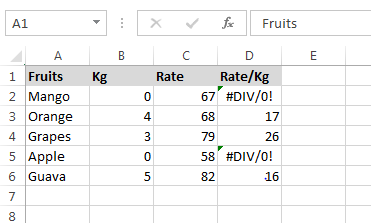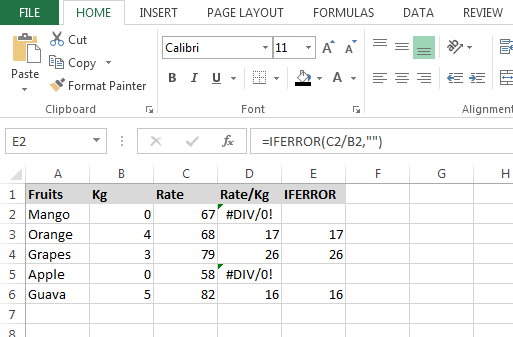# IFERROR Function in Microsoft Excel

In this article, we will learn how and where we can use Excel formula IFERROR.

IFERROR- This function is used when we get any error by using another formula or by doing the any calculation

The Syntax of IFERROR function: =IFERROR(Value,value_if_error)

Let’s take an example and understand how we can use Excel IFERROR formula.

We have fruits data in range A1:D6. Column A contains Fruits Name, Column B contains Kilograms, Column C contains Rate and in column D, we have returned the amount per kilogram.Where kilogram is zero the formula is giving #DIV/0! Error. We need a formula that can produce the result as 0 if the kilogram is zero, else show the rate per kilogram.

• Enter the formula in cell E2.
• =IFERROR(C2/B2,"")
• Press Enter on your keyboard.
• Copy the same formula in the rest of the cells.
• The function will return only value including 0.By Using IFERROR formula, we can ignore error while calculation in Microsoft Excel.

Terms and Conditions of use

The applications/code on this site are distributed as is and without warranties or liability. In no event shall the owner of the copyrights, or the authors of the applications/code be liable for any loss of profit, any problems or any damage resulting from the use or evaluation of the applications/code.